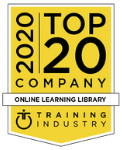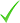# Mathematical and Computational Methods

edX
Training overview
Professional Course
Virtual Classroom
18 weeks
 From 249 USD
﻿
Start dates
Online
249 USD

## Mathematical and Computational Methods

Physicists use math all of the time in nearly everything that they work on. Hence, it is critical that you become efficient in being able to use more advanced math to enable you to work on more advanced physics courses. Most of you are currently what I would call a technician at math. You are able to perform the required manipulations to find and simplify answers. But, in many cases, you are missing the deeper understanding to help you interconnect different math topics and for you to be able to apply the relevant math for whatever problem you will face, based on your knowledge of how the different math topics inter-relate. On the other hand, a practitioner is someone who not only is technically adept at performing math calculations, but also have the insight and deeper understanding to know how to recognize what math applies to what problem. They understand how math is interconnected and recognize that math involves a handful of simple ideas that repeat. They are able to re-derive important formulas from basic principles or know precisely where to look them up and use them.

The goal of this class is to transform you from a math technician to a math practitioner. Mathematicians take this one step further and actually create new math. We will not focus on how to do that at all in this class.

Want to find out more?

None

## Training content

• Be able to apply techniques of calculus (learned in the first three semesters of a calculus sequence) to solve problems that arise in physics.
• Derive and use the geometric series in calculations.
• Manipulate power series expressions and employ them in physics contexts.
• Calculate Taylor polynomials/series of common functions and use them in approximating functions
• Follow the development for how one integrates polynomials, rational functions of polynomials, square roots of quadratics, rational functions with square roots of quadratics, and why the procedure cannot solve integrals with square roots of quartics.
• Solve integrals via parametric methods (differentiating under the integral sign) including techniques for introducing the parameter into the integrand
• Set up and integrate multidimensional integrals with variable mass density and for moments of inertia.
• Solve problems in multivariable integrals via the different integral theorems
• Solve Laplace's equation for simple geometries
• Compute the curl or divergence of a vector field and the gradient of a scalar function
• Solve complex numbers arithmetic problems
• Use calculus of residues to solve integrals
• Use row reduction to solve simultaneous linear equations
• Calculate the determinant, inverse, and eigenvalues of matrices
• Construct abstract vector spaces using the definitions of a vector space and generalized inner products
• Solve any first-order linear differential equation
• Solve the six classes of nonlinear first-order differential equations
• Solve higher-order differential equations with constant coefficients (homogeneous and inhomogeneous)
• Construct representations of curves in three dimensions using the Frenet-Serret apparatus
• Construct Fourier series of periodic functions

## Course delivery details

This course is offered through Georgetown University, a partner institute of EdX.

7-10 hours per week

## Costs

• Verified Track -\$249
• Audit Track - FreeedX For Business helps leading companies upskill their labor forces by making the world’s greatest educational resources available to learners across a wide variety of in-demand fields. edX For Business delivers high-quality corporate eLearning to train and engage your employees...

Read more and show all training delivered by this supplier

## Contact this provider

Fill out your details to find out more about Mathematical and Computational Methods.Contact the providerGet more informationRegister your interest

Country *

## edX

141 Portland Street
02139 Cambridge Massachusetts

Show phone number

Request Information

Have a question about this course? Fill out this form and the provider will get in touch with you shortly

View again
Supplier Directory